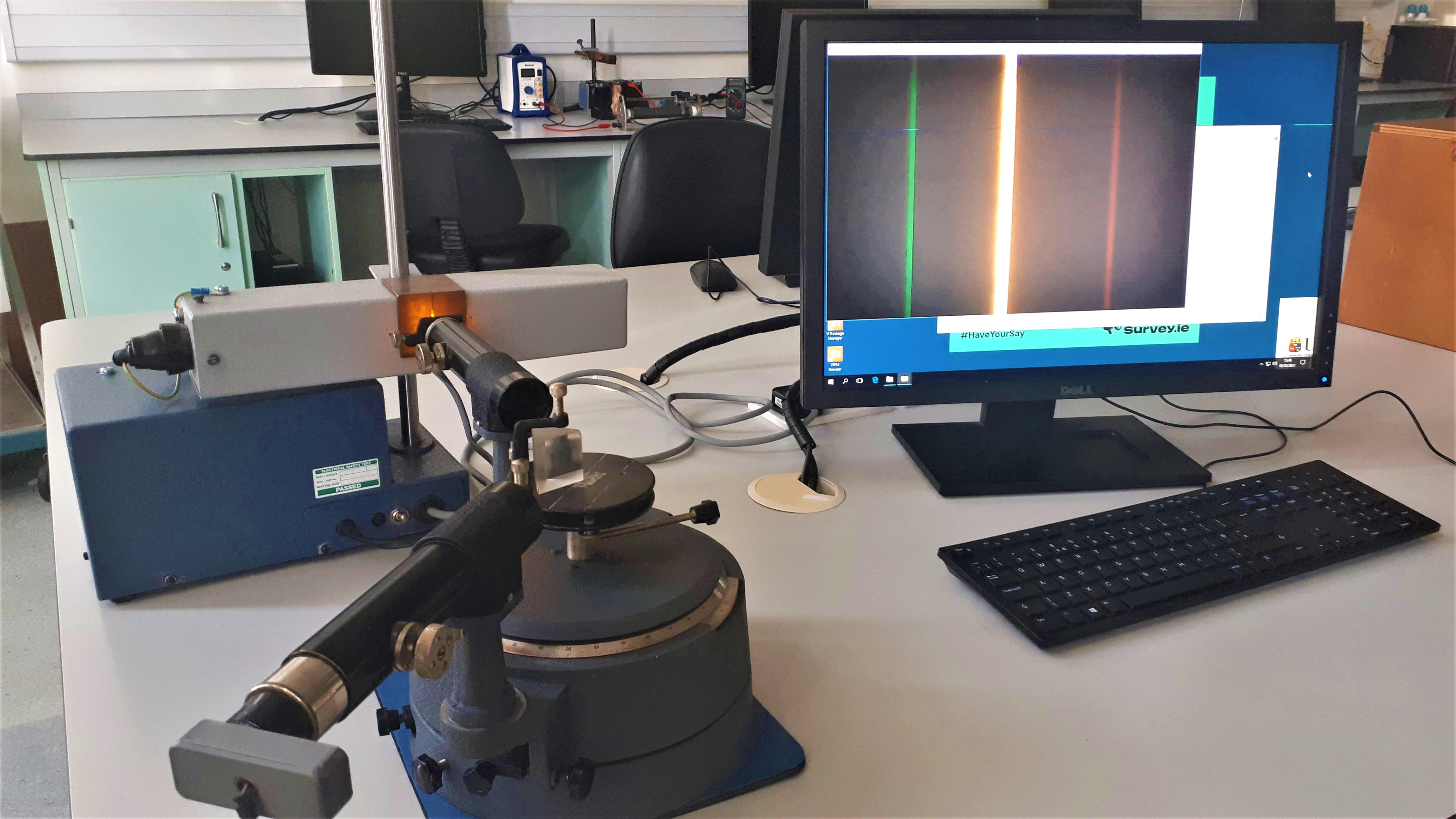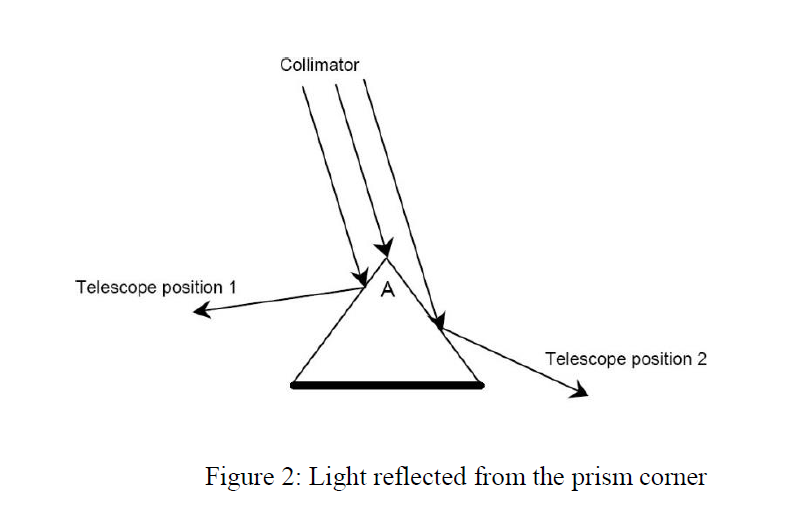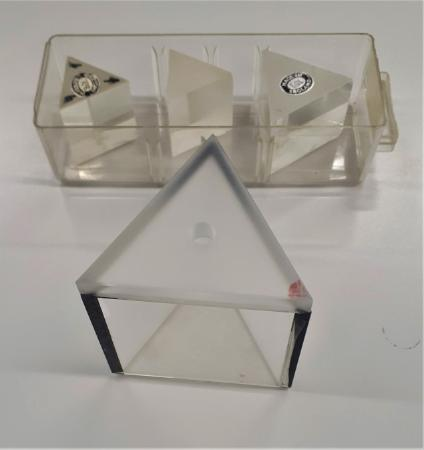# Determination of Refractive Index using a Prism

Prisms are solid glass optics that are ground and polished into geometrical and optically significant shapes. The angle, position, and number of surfaces help define the type and function. An ordinary triangular prism can separate white light into its constituent colours, called a spectrum. Each colour, or wavelength, making up the white light is bent, or refracted, a different amount; the shorter wavelengths (those toward the violet end of the spectrum) are bent the most, and the longer wavelengths (those toward the red end of the spectrum) are bent the least.

In this experiement a prism is used in conjunction with a spectrometer to analyse the spectral components in sodium light. The strongest line is at 589 nm (yellow)  and the other spectral lines that can be seen are mainly a green and a red line at the side of yellow line, plus two other transitions in the blue side of the spectrum. Students use the spectrometer to characterise the prisms in two ways.1. Measurement of the Prism Angle

First students measure the angle of the edges of the prism. This is achieved by shining a lsit of the sodium light directly onto the edge of the prism. The beam will be reflected off both sides of the prism (each acting like a plane mirror) so two images of the slit can be observed. By measuring the angle between each reflection and dividing it in two the students can get the angle A of the prism edge.2. Measurement of the Refractive Index of Glass

Using the physics phenomena of dispersion, which is the seperation of light into its seperate colours as it passes through an optical element, students can determine the refractive index of glass. As each colour is bent a different amount depending on the refractive index of glass students can use this property to measure the refractive index of the glass used to create the prism.3. Measurement of the Refractive Index of Water and Alcohol

Liquids also have a refractive index which can be measured. One method of doing this is to fill a hollow prism with the liquid to be measured and to carry out the experiemnts as above. The hollow prism used can be seen in the picture above.

if you want to explore the refeaction of light through a prism try this online lab: Refraction through a Prism

## Department of Physics

Roinn na Fisice

Room 213 (Physics Office), 2nd floor, Kane Science Building, University College Cork, Ireland.,

Top